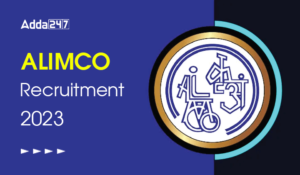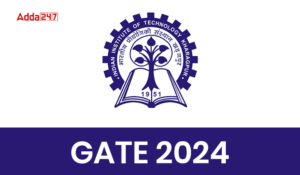Engineering Jobs   »   Mechincal Engineering quizs   »   UPPSC Lecturer Recruitment 2021, UPPSC Lecturer...

# UPPSC Lec’21 ME: Daily Practices Quiz. 12-Oct-2021

Quiz: Mechanical Engineering
Exam: UPPSC Lecturer
Topic: Industrial
Each question carries 1 mark
Negative marking: 1/4 mark
Time: 6 Minutes

Q1. The variance for critical path a-b-c-d-e is
a → b = 4, b → c = 16, c → d = 4, d → e = 1
The standard deviation of the critical path a → e is:
(a) 3
(b) 4
(c) 5
(d) 6

Q2. Dummy activities are
(a) facilitate computation of slacks
(b) satisfy precedence requirements
(c) determine project completion time
(d) avoid use of resources

Q3. Which one of the following is assumed for timing the activities in PERT network?
(a) α distribution
(b) β distribution
(c) Binomial distribution
(d) Erlangian distribution

Q4. In an assembly line, what is the balance delay?
(a) Line efficiency × 100
(b) 100 – Line efficiency (in percentage)
(c) (Line efficiency)/100
(d) None of the above

Q5. In single server queuing model if arrival rate is λ and service rate is μ, then what is the probability of the system being idle?
(a) λ/μ
(b) μ/λ
(c) 1 – λ/μ
(d) ((1-λ)/μ)

Q6. In the Kendall’s notation for representing queuing models the first position represents
(a) Probability law for the arrival
(b) Probability law for the service
(c) Number of channels
(d) Capacity of the system

Solutions

S1. Ans.(c)
Sol. Let V be the variance
So V_1=4,V_2=6,V_3=4,V_4=1
Standard deviation of critical path a → e is √(V_1+V_2+V_3+V_4 )
SD = √(4+6+4+1) = √25 = 5
S2. Ans.(b)
Sol. Dummy activity is used to maintain the logical sequence. It is used in network to satisfy precedence requirement and it does not consume time or resources.

S3. Ans.(b)
Sol. In PERT the distribution curve for the time taken i.e., optimistic time, most likely time and pessimistic time, to complete each activity resemble a β distribution.

S4. Ans.(b)
Sol. Balance Delay(D) = 1 – (Total Work content )/((Number of Cycles)× (Cycle time))=1-T_WC/(nT_C )
Line efficiency = T_WC/(nT_C )
So, D = 100 – Line efficiency (in percentage)

S5. Ans.(c)
Sol. if arrival rate is λ and service rate is μ
Then, Probability of utilization or channel efficiency =λ/μ
∴ Probability of idleness = 1 –λ/μ

S6. Ans.(a)
Sol. Kendall’s Lee notation
▭(a/b/c∶d/e/f)
a: Probability distribution of arrival.
b: Probability distribution of service.
c: Number of servers.
d: Service discipline (FCFS, FCLS).
e: System size.
f: Calling source population.

Sharing is caring!

•GATE Result 2023 Out, Download Result, C...
•GATE 2023 Admit Card Out, Download Link ...
•AP EAMCET Results 2023 Out Soon, Direct ...
•NMRC Recruitment 2023 Notification Out f...
•TSSPDCL AE Result 2023 Out, Download PDF...
•SECR Recruitment 2023 Out, Apply Online ...
•ALIMCO Recruitment 2023 Out, Apply For 1...
•GATE 2024, Registration, Notification, A...